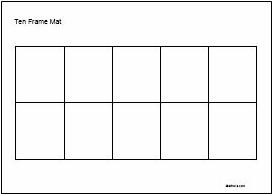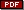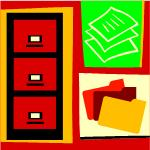># Using Place Value Math Mats

## Ten-Frame MatMath mats help students organize materials in a mathematically meaningful way. They may also serve as prompts to reinforce mathematical concepts and skills. For example,ten-frame mat help young students organize objects by tens, reinforcing that important place value concept of our base-ten number system.

It is developmentally appropriate to have students begin with ungrouped materials (e.g. unifix cubes, beans, etc.) and place one object in each square of the ten frame to create a ten. Using this organizational mat promotes mathematical discourse. Do you have ten cubes? How many more do you need? Such discussion helps develop the concepts of place value, decomposition of numbers, addition, subtraction, etc.

## Double Ten-Frame MatNext, students use adouble ten-frame mat to model numbers from 1-20. Consistent use of this mat enables students to make tens and visualize the teen numbers as the set of numbers that come right after 10, or between 10 and 20.

The teen numbers are notoriously hard for kindergarten students. Using this double ten-frame mat reinforces the concept that the teen numbers are ten plus another smaller number. The double ten-frame mat helps students visually make this connection. Read more about helping students master teen numbers,

Finally, ask students to name the number represented and either write the number (if inserted in a sheet protector) or select the appropriate number card. This ties the numerals to the concrete representation and forms the basis for an effective understanding of place value.

## Tens-Ones MatLater on, students transition to thetens-ones mat with ten frames in the ones place to build upon the ten-frame skills and introduce the tens place. The use of the mat prompts students to look for tens and regroup, as necessary, to represent 2-digit numbers. Finally, asking students to write the correct digit in both the tens and the ones column helps students learn how our base-ten system works to build numbers.

Unifix cubes or other linking cubes are excellent manipulatives for this mat. Students may place individual cubes in each of the ten-frame squares. Once they have filled a ten-frame, they simply snap the cubes together to form a ten-train and move it to the tens column. Once again, this concretely supports the concept of regrouping ten ones to make a ten.

## Mathwire.com: Math Mats ResourcesThis series of pages is designed as a resource to teachers as they differentiate instruction for varied learners in the class. Each page discusses how to use the set of mats to develop mathematical understanding and support student growth.